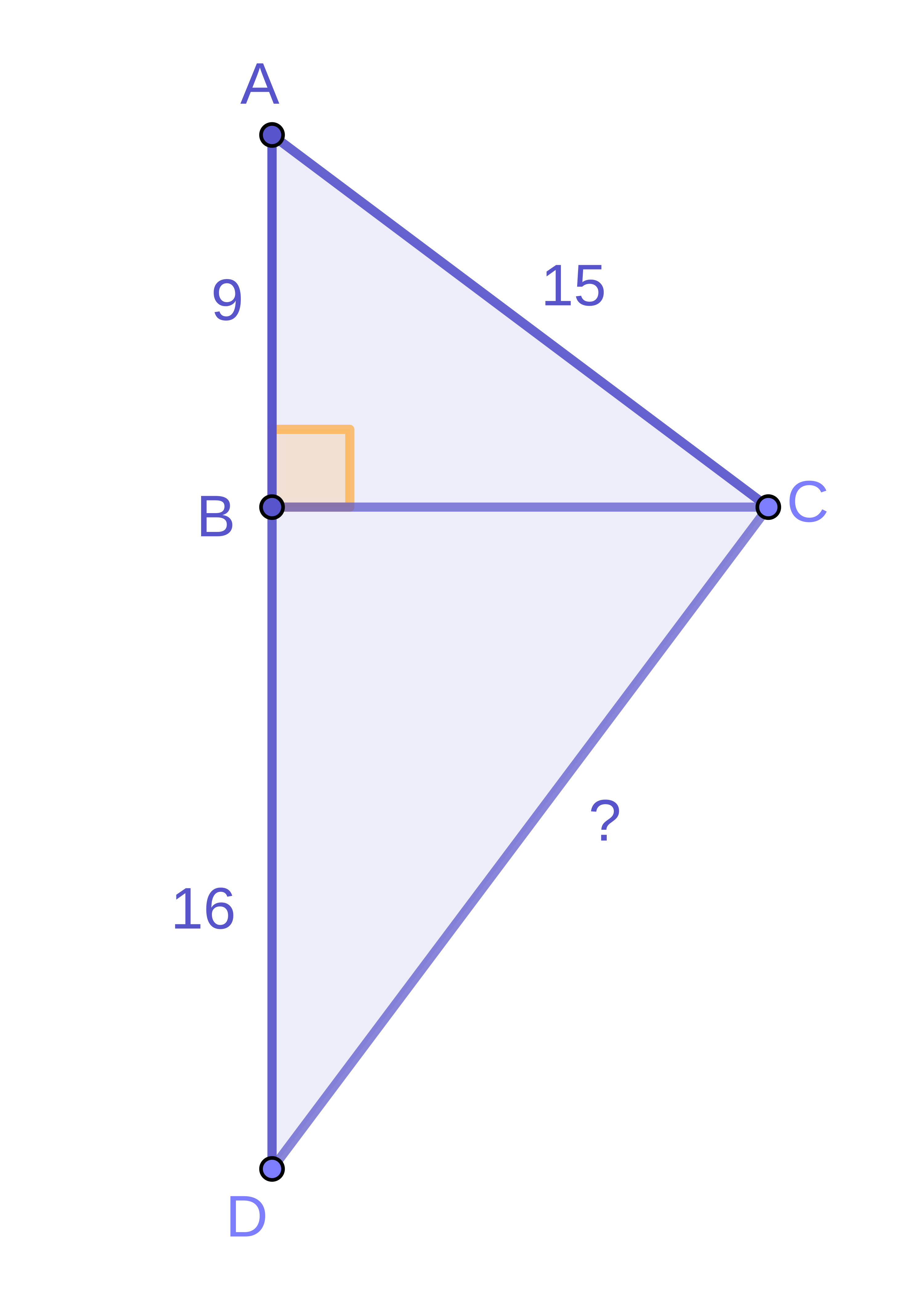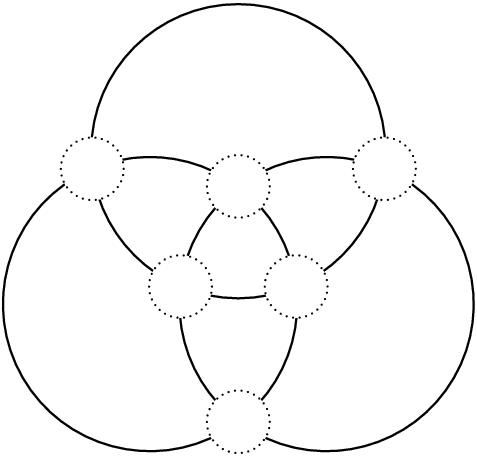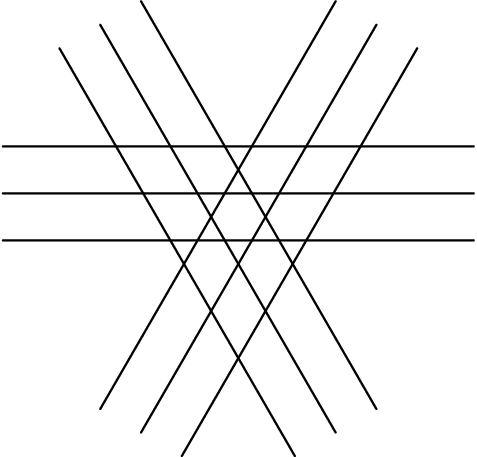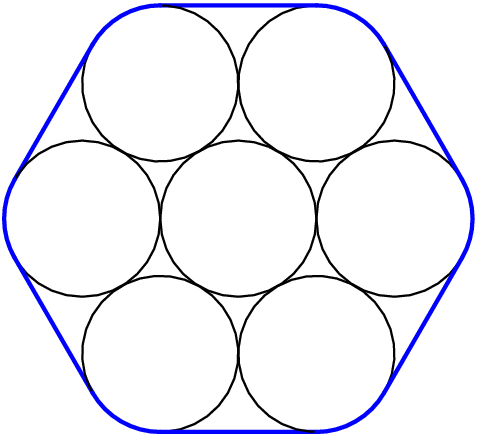##### Introduction: 자격 확인 시험

여러분이 너무 쉽지 않은 수업을 들으시면 좋겠습니다. 이 시험에서 70% 미만의 점수를 받는다면 이 강좌를 통해 새로운 내용을 배울 수 있습니다.

남은 시간:

1:00:00

1.

Five rectangles, $$A,B, C, D$$ and $$E$$ have integer side lengths. Rectangle $$A$$ has a width of $$10$$, rectangle $$B$$ has a width of $$12,$$ rectangle $$C$$ has a length of $$14,$$ rectangle $$D$$ has a length of $$28,$$ and rectangle $$E$$ has a length of $$20.$$

If all five rectangles have the same area $$K$$, what is the least possible value of $$K$$?

2.

What is the missing side length in the triangle below?3.

How many factors of the number $$648$$ are perfect squares?

4.

There are $$8$$ empty parking spaces in a row at a car dealership lot. Three identical cars drive into the parking lot, each of them choosing a random empty parking space to park in. Since the cars are new, they don't have license plates.

What is the probability that all $$3$$ cars are parked next to each other?

Your answer should be a fraction in simplest form with no spaces.

For example, if the answer were $$\frac{3}{2},$$ you would enter: $$3/2$$

5.

If $$a$$, $$b$$, and $$c$$ are numbers that satisfy:

\begin{align*} a^2b&=20\\ b^2c&=30\\ c^2a&=45, \end{align*}

then what is the value of $$abc$$?

6.

A scientist is studying a cell under a microscope. First, she uses a lens that magnifies 200× (that makes the length and width dimensions of the cell look 200 times longer), but the cell is still too small. If the scientist uses a 500× lens instead, how many times as much area will the cell appear to take up compared to when she uses the 200× lens?

Your answer should be a decimal rounded to the nearest hundredth, e.g. 1.21

7.

Three circles are drawn to intersect each other like a Venn Diagram, and there is a blank spot on each of the $$7$$ intersection points, as shown.Each blank spot is filled with one of these six numbers: $$2, 3, 4, 5, 6,$$ and $$7.$$ The sum of the numbers on each circle is the same. What is the sum of the numbers on one circle?

8.

How many triangles are in this figure?Triangles count even if they have other lines passing through them.

9.

At a school, every student likes either cats or dogs (but not both), and likes either apples or bananas (but not both). Also, $$\frac13$$ of the students who like cats also like apples, and $$\frac13$$ of the students who like apples also like dogs. Lastly, $$\frac34$$ of the students who like dogs also like bananas.

What percent of the students like bananas?

As shown below, $$7$$ circular pegs of the same radius are bundled together so that they are tangent to one another, and a string is wrapped around the outside. If the pegs' radii are all increased by an inch, how much longer would the string around the pegs be, in inches?